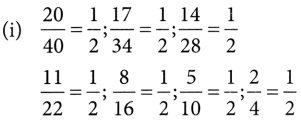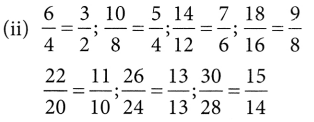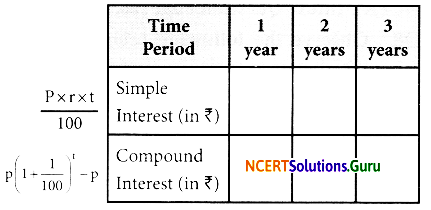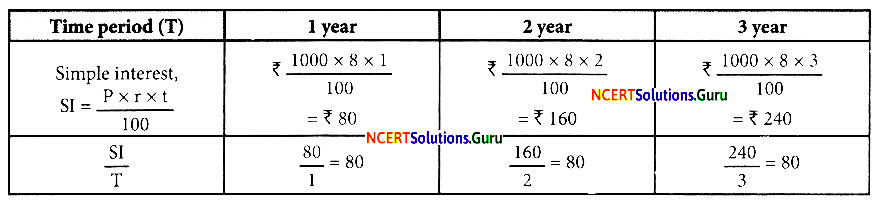# NCERT Solutions for Class 8 Maths Chapter 13 Direct and Inverse Proportions InText Questions

These NCERT Solutions for Class 8 Maths Chapter 13 Direct and Inverse Proportions InText Questions and Answers are prepared by our highly skilled subject experts.

## NCERT Solutions for Class 8 Maths Chapter 13 Direct and Inverse Proportions InText Questions

NCERT Intext Question Page No. 204

Question 1.
Observe the following tables and find if x and y are directly proportional.i. e. each ratio is the same
.’. x and y are directly proportionali. e. all the ratios are not the same x
and y are not directly proportional.i.e. all the ratios are not the same
x and y are not directly proportional.

Question 2.
Principal = ₹ 1000, Rate = 8% per annum. Fill in the following table and find which type of interest (simple or compound) change is in direct proportion with time period.Case of Simple Interest
[P = ₹ 1000, r = 8% p.a.]In each case the ratio is the same.
The simple interest changes in direct proportion with time period.
Case of Compound Interest [P = ₹ 1000, r = 8% p.a.]$$\frac{\mathrm{CI}}{\mathrm{T}}$$ is not the same in each case.
∴ The compound interest does not change in direct proportion with time period.NCERT Intext Question Page No. 211

Question 1.
Observe the following tables and find which pair of variables (here x and y) are in the inverse proportion.(i) x1y1 = 50 x 5 = 250
x2y2 = 40 x 6 = 240
x1y1 ≠ x2y2
Hence, x and y are not in inverse proportion.

(ii) x1y1 = 100 x 60 = 6000
x2y2 = 200 x 30 = 6000
x3y3 = 300 x 20 = 6000
x4y4 = 400 x 15 = 6000

x1y1 = x2y2 = x3y3 = x4y4
Hence x and y are in inverse proportion.
(iii) x1y1 = 90 x 10 = 900
x2y2 = 60 x 15 = 900
x3y3 = 45 x 20 = 900
x4y4 = 30 x 25 = 750
x1y1 = x2y2 = x3y3 ≠ x4y4
.’. x and y are not in inverse proportion.
Note: (1) When two quantities x and y are in direct proportion (or vary directly), they are also written is x ∝ y.
(2) When two quantities x and y are in inverse proportion (or very inversely), they are also written as x ∝ $$\frac{1}{y}$$.

error: Content is protected !!